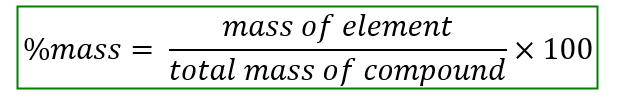# Problem: Calculate the following:Mass % of I in strontium periodate

###### FREE Expert Solution

Mass or weight percent is the percentage of a given element in a compound.Step 1: Write the molecular formula of strontium periodate.

Strontium is a metal from group 2A with an elemental symbol Sr. Metals from group 2A has the charge +2 when acting as a cation.

Periodate is a polyatomic anion with the formula IO4-.

94% (255 ratings)###### Problem Details

Calculate the following:

Mass % of I in strontium periodate